Quantitative MCQ - 9

# Quantitative MCQ - 9

Test Description

## 30 Questions MCQ Test Quantitative Aptitude for Competitive Examinations | Quantitative MCQ - 9

Quantitative MCQ - 9 for Quant 2022 is part of Quantitative Aptitude for Competitive Examinations preparation. The Quantitative MCQ - 9 questions and answers have been prepared according to the Quant exam syllabus.The Quantitative MCQ - 9 MCQs are made for Quant 2022 Exam. Find important definitions, questions, notes, meanings, examples, exercises, MCQs and online tests for Quantitative MCQ - 9 below.
Solutions of Quantitative MCQ - 9 questions in English are available as part of our Quantitative Aptitude for Competitive Examinations for Quant & Quantitative MCQ - 9 solutions in Hindi for Quantitative Aptitude for Competitive Examinations course. Download more important topics, notes, lectures and mock test series for Quant Exam by signing up for free. Attempt Quantitative MCQ - 9 | 30 questions in 30 minutes | Mock test for Quant preparation | Free important questions MCQ to study Quantitative Aptitude for Competitive Examinations for Quant Exam | Download free PDF with solutions
 1 Crore+ students have signed up on EduRev. Have you?
Quantitative MCQ - 9 - Question 1

### In how many different ways can the letters of the word ‘PRAISE’ be arranged?

Detailed Solution for Quantitative MCQ - 9 - Question 1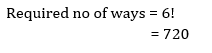Quantitative MCQ - 9 - Question 2

### Raju got married 8 years ago. His present age is 6/5 times his age at the time of his marriage. Raju’s sister was 10 years younger to him at the time of his marriage. The present age of Raju’s sister is:

Detailed Solution for Quantitative MCQ - 9 - Question 2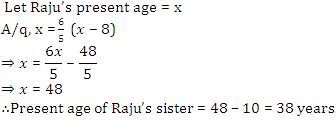Quantitative MCQ - 9 - Question 3

### Raman borrowed some money at the rate of 6% p.a. for the first three years, 9% p.a. for the next five years and 13% p.a. for the period beyond eight years. If the total interest paid by him at the end of eleven years is Rs. 8160, how much money did he borrow?

Detailed Solution for Quantitative MCQ - 9 - Question 3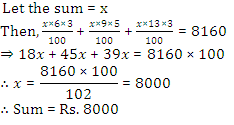Quantitative MCQ - 9 - Question 4

My brother is 3 years elder to me. My father was 28 years of age when my sister was born while my mother was 26 years of age when I was born. If my sister was 4 years of age when my brother was born, then, what was the age of my father and mother respectively when my brother was born?

Detailed Solution for Quantitative MCQ - 9 - Question 4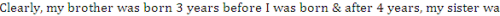born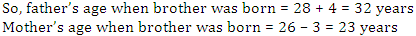Quantitative MCQ - 9 - Question 5

A solid diamond falls and breaks into three pieces whose weights are in 2 : 3 : 4. The value of each piece is directly proportional to the square of their weights. Given that the value of solid diamond is Rs. 24300. What was his loss due to breakage?

Detailed Solution for Quantitative MCQ - 9 - Question 5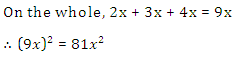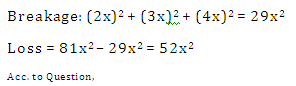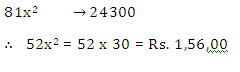Quantitative MCQ - 9 - Question 6

The vehicle of Mr. Ghosh needs 30% more fuel at the speed of 75 kmph than it needs at the speed of 50 kmph. At a speed of 50 kmph, Mr Ghosh can go to a distance of 195 kms. At the speed of 75 kmph, what distance he will travel?

Detailed Solution for Quantitative MCQ - 9 - Question 6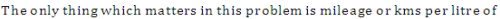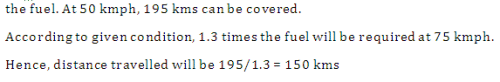Quantitative MCQ - 9 - Question 7

Direction (7-11): Study the graph to answer the questions.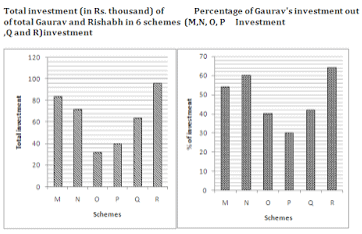Scheme M offers simple interest at a certain rate of internest (percent per annum). If the difference between the interest earned by Gaurav and Rishabh from scheme M after 4 yr is Rs. 4435.20, what is the rate of interest (percent per annum)?

Detailed Solution for Quantitative MCQ - 9 - Question 7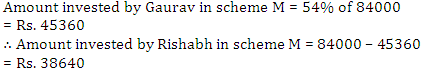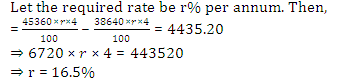Quantitative MCQ - 9 - Question 8

Direction (7-11): Study the graph to answer the questions.What is the respective ratio between total amount invested by Gaurav in schemes O and Q together and total amount invested by Rishabh in the same scheme together?

Detailed Solution for Quantitative MCQ - 9 - Question 8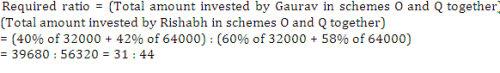Quantitative MCQ - 9 - Question 9

Direction (7-11): Study the graph to answer the questions.If scheme O offers compound interest (compounded annually) at 12% per annum, then what is the difference between interest earned by Gaurav and Rishabh from scheme O after 2 yr?

Detailed Solution for Quantitative MCQ - 9 - Question 9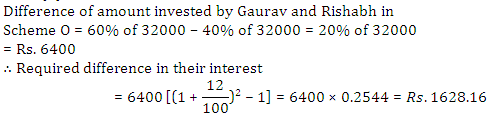Quantitative MCQ - 9 - Question 10

Direction (7-11): Study the graph to answer the questions.Rishabh invested in scheme R for 4 yr. If scheme R offers simple interest at 7% per annum for the first two years and then compound interest at 10% per annum (compound annually) for the 3rd and 4th year, then what will be the interest earned by Rishabh after 4 yr?

Detailed Solution for Quantitative MCQ - 9 - Question 10Quantitative MCQ - 9 - Question 11

Direction (7-11): Study the graph to answer the questions.Amount invested by Gaurav in another scheme S is equal to the amount invested by him in scheme N. The rate of interest per annum of schemes S and N are same. The only difference is scheme S offers compound interest (compounded annually), whereas the scheme N offers simple interest. If the difference between the interest earned by Gaurav from both the schemes after 2 yr is Rs. 349.92, then what is the rate of interest?

Detailed Solution for Quantitative MCQ - 9 - Question 11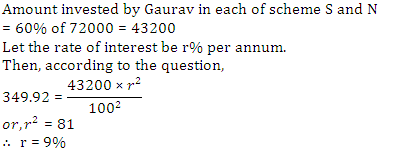Quantitative MCQ - 9 - Question 12

Directions (12-16): Study the following table carefully and answer the following questions related to it.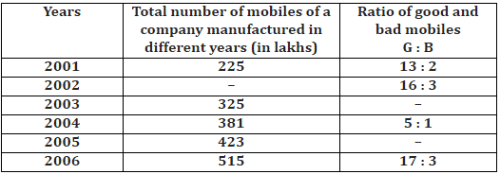Note: There are some missing data in the table given above. If there is any requirement of these missing data in any question, find them and then proceed.

What is the average number of good mobiles manufactured by company in the years 2001, 2003 and 2004 together if total number of good mobiles manufactured by company in the year 2003 was 273 lakhs?

Detailed Solution for Quantitative MCQ - 9 - Question 12Quantitative MCQ - 9 - Question 13

Directions (12-16): Study the following table carefully and answer the following questions related to it.Note: There are some missing data in the table given above. If there is any requirement of these missing data in any question, find them and then proceed.

If the bad mobiles in the year 2001 were (200/3)% of bad mobiles in the year 2002, what is the total number of good mobiles in the years 2001 and 2002 together?

Detailed Solution for Quantitative MCQ - 9 - Question 13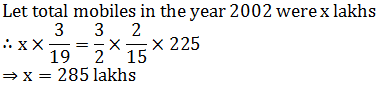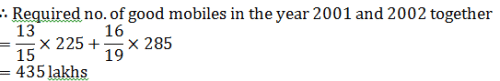Quantitative MCQ - 9 - Question 14

If the difference between total number  of good mobiles in the year 2004 and 2005 was 11.5 lakhs then what was the total number of bad mobiles in the year 2005?

Detailed Solution for Quantitative MCQ - 9 - Question 14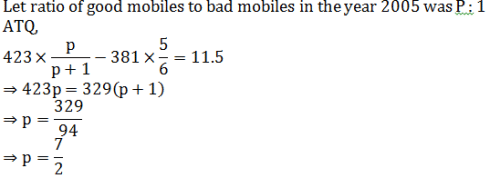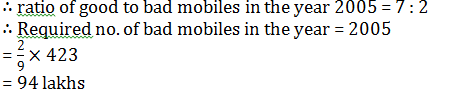Quantitative MCQ - 9 - Question 15

Directions (12-16): Study the following table carefully and answer the following questions related to it.Note: There are some missing data in the table given above. If there is any requirement of these missing data in any question, find them and then proceed.

What is the ratio of number of good mobiles in the year 2004 to the number of good mobiles in the year 2006?

Detailed Solution for Quantitative MCQ - 9 - Question 15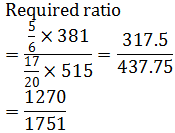Quantitative MCQ - 9 - Question 16

Directions (12-16): Study the following table carefully and answer the following questions related to it.Note: There are some missing data in the table given above. If there is any requirement of these missing data in any question, find them and then proceed.

The number of bad mobiles manufactured in year 2001 are approximately what percent more or less than the number of bad mobiles manufactured in the year 2003? (Use the missing data found in Q1. If required)

Detailed Solution for Quantitative MCQ - 9 - Question 16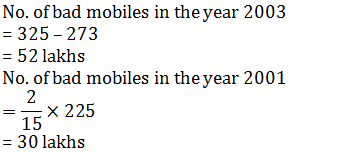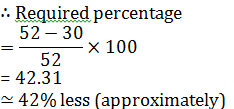Quantitative MCQ - 9 - Question 17

A machine depreciates in value each year at the rate of 10% of its previous value. However, every second year there is some maintenance work so that in that particular year, depreciation is only 5% of its previous value. If at the end of the fourth year, the value of the machine stands at Rs 1,46,205, then find the value of machine at the start of the first year.

Detailed Solution for Quantitative MCQ - 9 - Question 17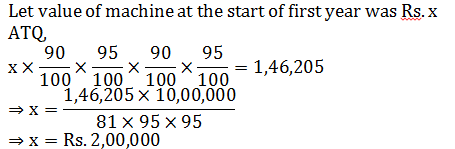Quantitative MCQ - 9 - Question 18

In the university examination last year, Rajesh scored 65% in English and 82% in History. What is the minimum percent he should score in Sociology, which is out of 50 marks (if English and History were for 100 marks each), if he aims at getting 78% overall?

Detailed Solution for Quantitative MCQ - 9 - Question 18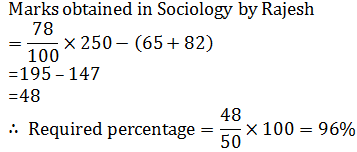Quantitative MCQ - 9 - Question 19

A sold a table to B at a profit of 20%. B sold the same table to C for Rs 75 thereby making a profit of 25%. Find the price at which A bought the table from X.

Detailed Solution for Quantitative MCQ - 9 - Question 19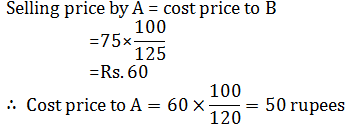Quantitative MCQ - 9 - Question 20

A watch dealer sells watches at Rs 600 per watch. However, he is forced to give two successive discounts of 10% and 5% respectively. However, he recovers the sales tax on the net sale price from the customer at 5% of the net price. What price does a customer have to pay him to buy the watch?

Detailed Solution for Quantitative MCQ - 9 - Question 20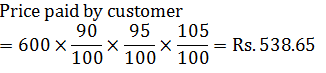Quantitative MCQ - 9 - Question 21

A pet shop owner sells two puppies at the same price. On one he makes a profit of 20% and on the other he suffers a loss of 20%. Find his loss or gain per cent on the whole transaction.

Detailed Solution for Quantitative MCQ - 9 - Question 21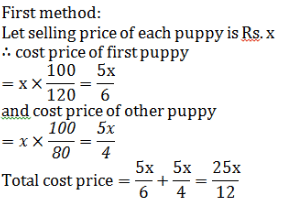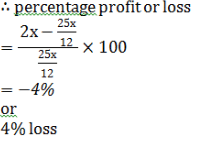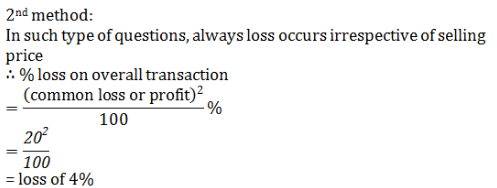Quantitative MCQ - 9 - Question 22

Directions (22-25): What will come in place of question mark (?) in the questions that follow:

2¾÷3¾× (125/16)×256=?

Detailed Solution for Quantitative MCQ - 9 - Question 22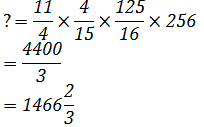Quantitative MCQ - 9 - Question 23

Directions (22-25): What will come in place of question mark (?) in the questions that follow:

9999 ÷ 99 × 73 + 251 = ?

Detailed Solution for Quantitative MCQ - 9 - Question 23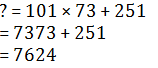Quantitative MCQ - 9 - Question 24

Directions (22-25): What will come in place of question mark (?) in the questions that follow:

36% of 545 + 32% of 215 – 47% of 1300 = ?

Detailed Solution for Quantitative MCQ - 9 - Question 24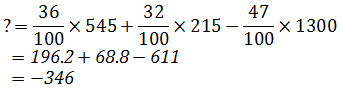Quantitative MCQ - 9 - Question 25

Directions (22-25): What will come in place of question mark (?) in the questions that follow:

√1225÷ ∛343× 45% of 760 = ?

Detailed Solution for Quantitative MCQ - 9 - Question 25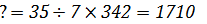Quantitative MCQ - 9 - Question 26

In a test, a candidate secured 336 marks out of maximum marks x. If the maximum marks x were converted into 400 marks, he would secure 192 marks. What were the maximum marks of the test?

Detailed Solution for Quantitative MCQ - 9 - Question 26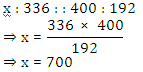Quantitative MCQ - 9 - Question 27

The ratio of the age of a man and his wife is 4 : 3. After 4 years this ratio will be 9 : 7. If at the time of the marriage, the ratio was 5 : 3, then how many years ago they were married?

Detailed Solution for Quantitative MCQ - 9 - Question 27

Lets present ages of a man and his wife is 3x and 4x years respectively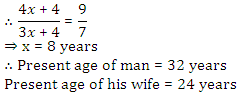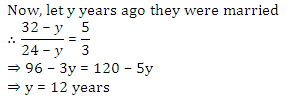Quantitative MCQ - 9 - Question 28

Tea worth Rs. 126 per kg and Rs. 135 per kg are mixed with a third variety in the ratio 1 :  1 : 2. If the mixture is worth Rs. 153 per kg, then the price of third variety per kg will be

Detailed Solution for Quantitative MCQ - 9 - Question 28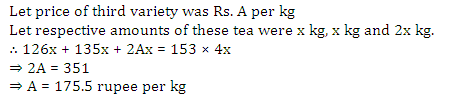Quantitative MCQ - 9 - Question 29

Several litre of Acid were drawn off a 54-litre vessel full of Acid and an equal amount of water added. Again the same volume of the mixture was drawn off and replaced by water as a result the vessel contained 24 litres of pure acid. How much of the Acid was drawn off initially?

Detailed Solution for Quantitative MCQ - 9 - Question 29

Lets initialy x liter of water drawn off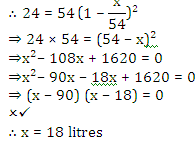Quantitative MCQ - 9 - Question 30

12 men take 36 days to do a work while 12 women complete 3/4th of the same work in 36 days. In how many days 10 men and 8 women together will complete the same work?

Detailed Solution for Quantitative MCQ - 9 - Question 30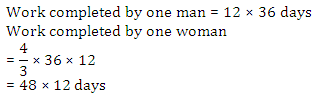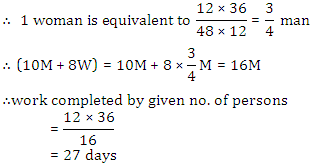## Quantitative Aptitude for Competitive Examinations

33 videos|34 docs|140 tests
 Use Code STAYHOME200 and get INR 200 additional OFF Use Coupon Code
Information about Quantitative MCQ - 9 Page
In this test you can find the Exam questions for Quantitative MCQ - 9 solved & explained in the simplest way possible. Besides giving Questions and answers for Quantitative MCQ - 9, EduRev gives you an ample number of Online tests for practice

## Quantitative Aptitude for Competitive Examinations

33 videos|34 docs|140 tests